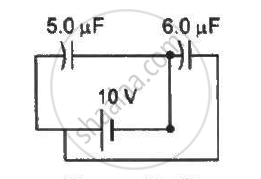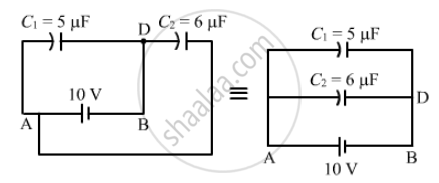Department of Pre-University Education, Karnataka course PUC Karnataka Science Class 12
Share

# P Find the Charge Supplied by the Battery in the Arrangement Shown in Figure . - Physics

ConceptCapacitors and Capacitance

#### Question

Find the charge supplied by the battery in the arrangement shown in figure.#### Solution

The equivalent circuit for the given case can be drawn as :-It can be observed that capacitors C1 and C2 are in parallel.
Therefore, the equivalent capacitance is given by

Ceq = C1 C2
= 5 + 6 = 11 μF

Now ,

Charge supplied by the battery = (Ceq)(V) = (11 μF) × (10 V) = 110 μC

Is there an error in this question or solution?

#### Video TutorialsVIEW ALL 

Solution P Find the Charge Supplied by the Battery in the Arrangement Shown in Figure . Concept: Capacitors and Capacitance.
S